# How to use Excel VLOOKUP : Learn with ExamplesHow to        January 01, 2022         TILS Education       0       1.55K

## What is VLOOKUP?

VLOOKUP (V stands for ‘Vertical’) is an in-built function in excel for looking up a piece of information in a table or data set and extracting some corresponding data/information.

In other words, VLOOKUP looks for a given value in a vertical list / table array, and once it has found that value, it returns respective or corresponding value from specified column number.

## Usages of VLOOKUP?

VLOOKUP function is best suited for situations when you are looking for a matching data point in a column, and when the matching data point is found, you go to the right in that row and fetch a value from a cell which is a specified number of columns to the right.

## VLOOKUP Syntax

##### =VLOOKUP(lookup_value, table_array, col_index_num , [range_lookup])

As per above VLOOKUP formula, it takes 4 arugments

1. Lookup_value(Required) - this is the look-up value that we want to look up in the left-most column of a table.
2. Table_array(Required) - The table array is the data array that is to be searched. The VLOOKUP function searches in the left-most column of this array.
3. Col_index(Required) - specifying the column number of the table array from which we want to fetch the matching value.
4. Range_lookup(optional argument) - here you specify whether you want an exact match or an approximate match. If omitted, it defaults to TRUE
1. True – Approximate match, it means if exact match is not found, then it uses closest match below the lookup_value
2. False – Exact Match, it means if exact match is not found, then it will return error

## VLOOKUP EXAMPLE

We have a list with students’ roll number and marks of three subjects as per below screenshotNow if we want to display all three subjects’ marks by entering any specific roll number in cell G3, then we use VLOOKUP formula as mentioned below

=VLOOKUP(\$G\$3,\$A\$3:\$D\$17,2)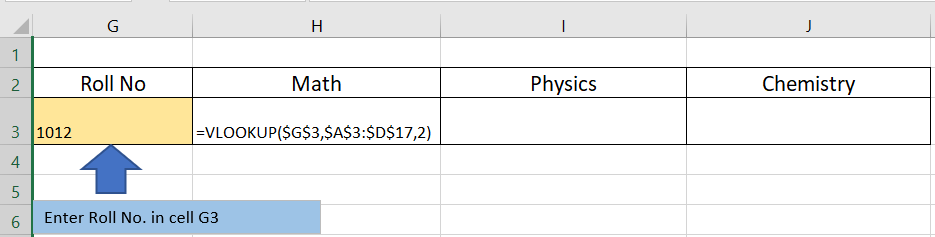Here, in above formula

1. We enter above formula in cell H3 to find Marks of Math Subject
2. First Argument (lookup_value) – is our roll no column i.e G3
3. Second Argument(table_array)- is our data array, in which we searched
4. Third Argument(col_index) – is our index number of table array, in above example we wrote 2 as index number, so it will return 2nd column value from table array i.e Math subject marks.

Similarly, we enter same formula in Cell I3 & J3 to find out other subjects marks by only changing column index number as per below image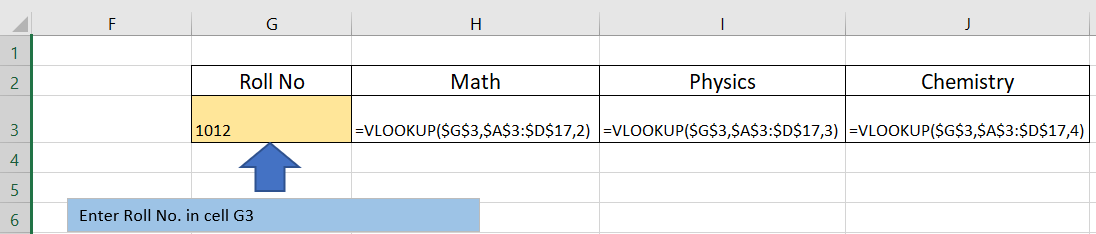Now, if we enter any roll number in cell G3, it will display all three subjects’ marks in respective cells. In below image we enter roll number 1012 in cell G3 and it will return all subject marks .## Excel VLOOKUP - things to remember!

• The VLOOKUP function cannot look at its left. It always searches in the leftmost column of the table array and returns a value from a column to the right.
• You can’t have duplicate values in the leftmost column of the lookup range. If the lookup column contains duplicate values, VLOOKUP will match with the first value only.
• The VLOOKUP function is case-insensitive, meaning that uppercase and lowercase characters are treated as equivalent. To distinguish the letter case, use case sensitive VLOOKUP formulas.
• Remember about the importance of the last parameter. Use TRUE for approximate match and FALSE for exact match.
• When searching for approximate match, make sure the data in the lookup column is sorted in ascending order.
• If the lookup value is not found, a #N/A error is returned.
• When referring to the lookup table, you want absolute cell references when you copy the VLOOKUP formula to other cells.#### TILS Education

The Institute of Languages & Skills (TILS) is an Educational Start-Up to root-up the biggest problem of India “Unemployability” even after having a dignified degree.

Welcome to the BlogzBite.com Informational Article Web Page.

###### Here At,Our aim is to provide you all the latest informational articles related to different niches, because we know knowledge is power and we want to be your medium of power.

### Popular Posts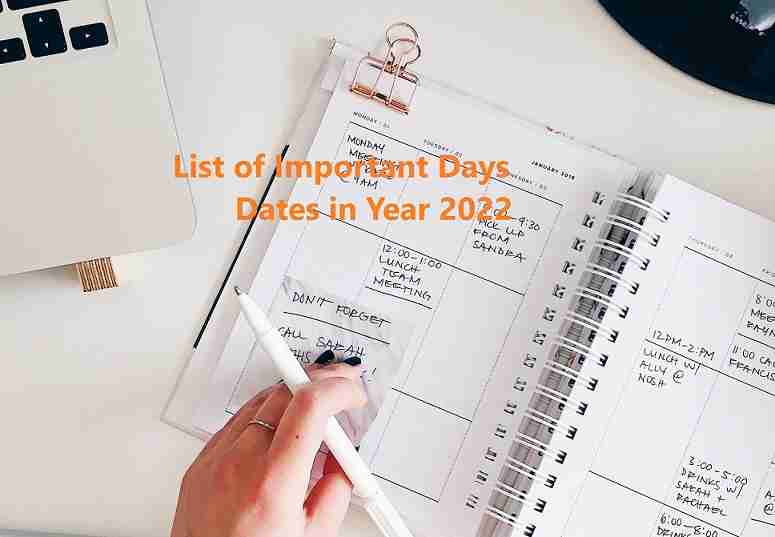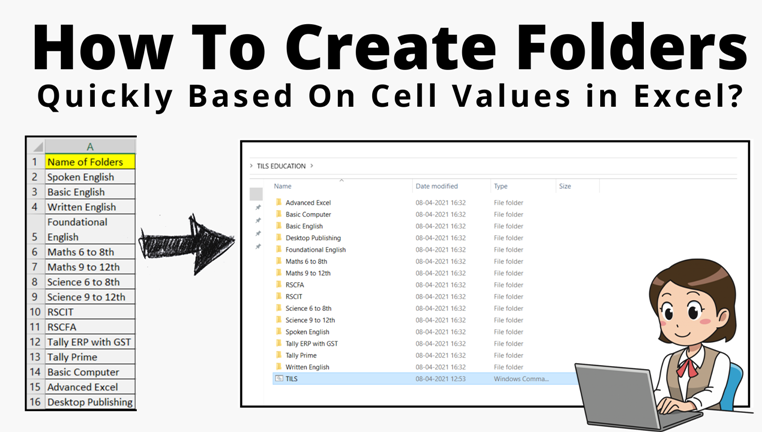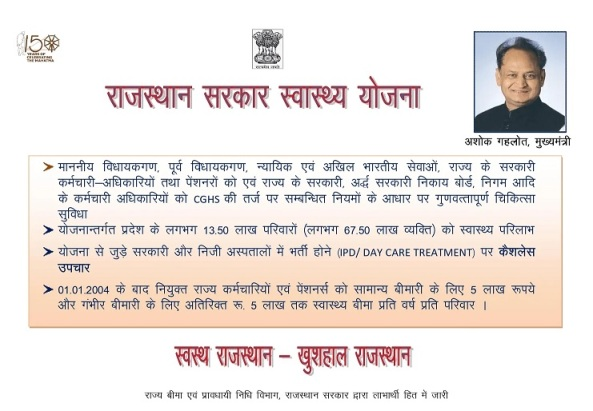Subscribe Us